Function Repository Resource:

# NetParallelOperator

Perform multiple operations on an input in a neural net

Contributed by: Sjoerd Smit
 ResourceFunction["NetParallelOperator"][{net1,net2,…}] represents a net with a single input and multiple outputs which correspond to applying the different networks to the input. ResourceFunction["NetParallelOperator"][<|out1→ net1,out2→net2,…|>] specifies that the output of neti should be linked to output port outi. ResourceFunction["NetParallelOperator"][spec, cat] uses a layer or net to combine the outputs into a single array again. ResourceFunction["NetParallelOperator"][spec,Automatic] catenates the outputs sequentially using CatenateLayer.

## Examples

### Basic Examples (2)

Define a net that computes the Sin, Cos and Tan of an input:

 In:=Out=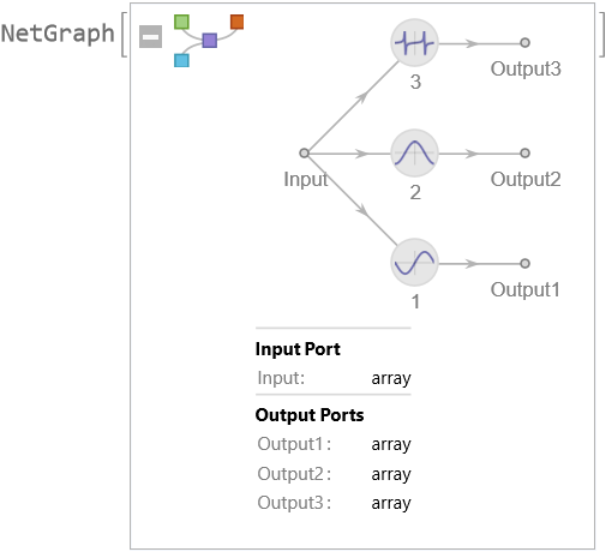Apply it to one or more values:

 In:=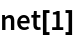Out=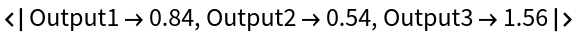In:=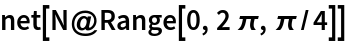Out=Specify custom names for the output ports:

 In:=Out=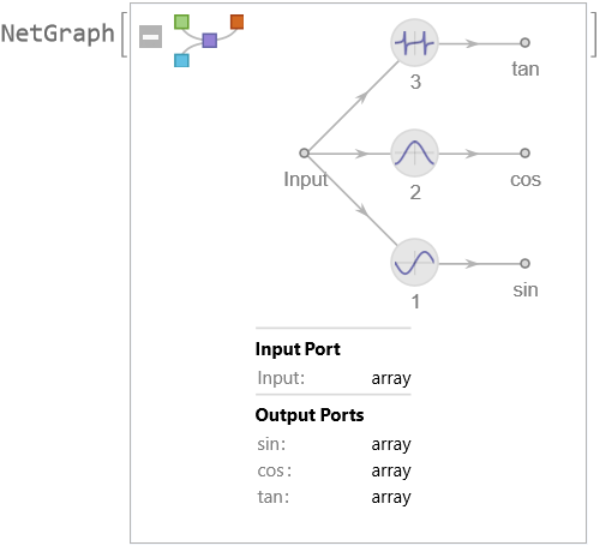In:=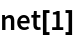Out=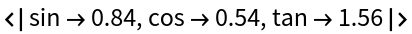### Scope (2)

Convert the output to a single array again by joining the results:

 In:=Out=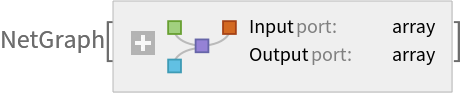In:=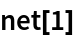Out=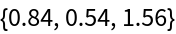In:=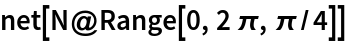Out=Use a different operation for combining the results into tuples:

 In:=Out=In:=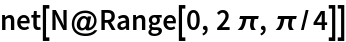Out=### Applications (5)

Create a network that can find a matrix with specific row and column sums while keeping the size of the elements as small as possible. NetParallelOperatorcan be used to calculate the required sums:

 In:=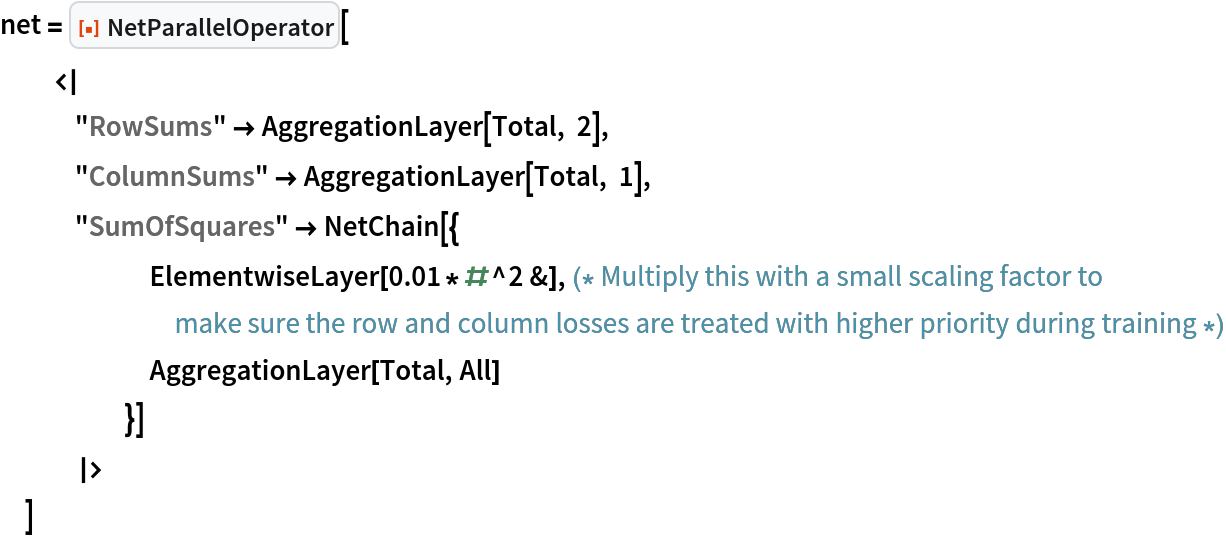Out=Define a training net with a learnable matrix:

 In:=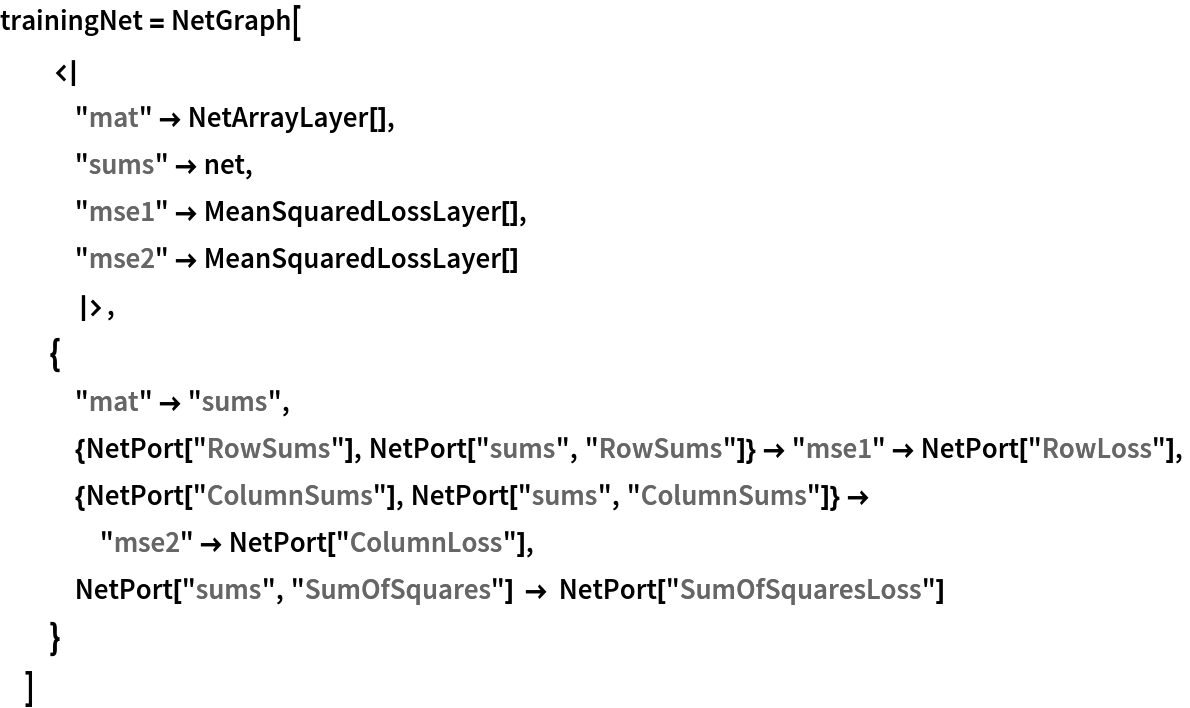Out=Train the net to find a matrix with the given row and column sums:

 In:=Out=Extract the matrix found by the model:

 In:=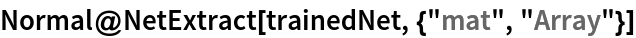Out=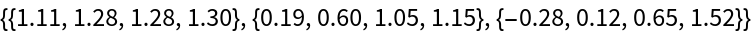Check the losses of the row and column deviations:

 In:=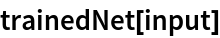Out=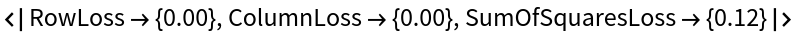Sjoerd Smit

## Version History

• 1.0.0 – 11 October 2022